# Texas Go Math Kindergarten Module 14 Assessment Answer Key

Refer to our Texas Go Math Kindergarten Answer Key Pdf to score good marks in the exams. Test yourself by practicing the problems from Texas Go Math Kindergarten Module 14 Assessment Answer Key.

## Texas Go Math Kindergarten Module 14 Assessment Answer Key

Concepts and Skills

Question 1.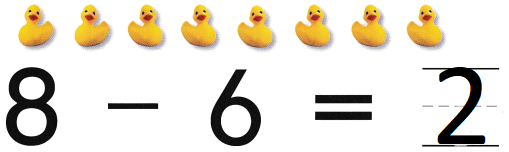Explanation:
There are 8 ducks.
Six ducks swim away.
8 – 6 = 2
so, 2 ducks are left

Question 2.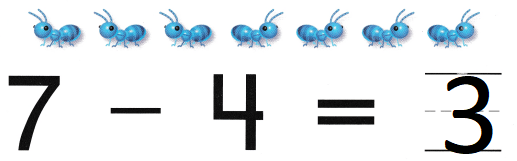Explanation:
There are 7 ants Four ants walk away
7 – 4 = 3
so, 3 ants are left

Question 3.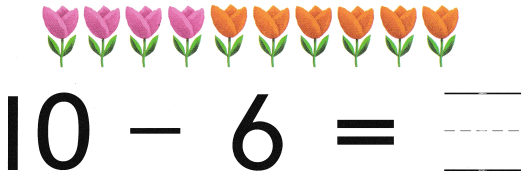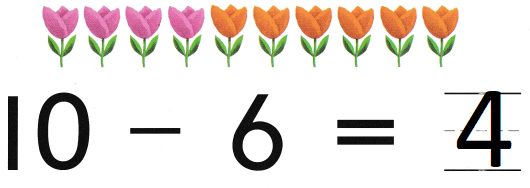Explanation:
There are 10 flowers.
Six of the flowers are orange.
The rest are pink.
10 – 6 = 4
so, 6 flowers are pink

Directions
1. There are 8 ducks. Six ducks swim away. How many ducks are left? Write the number. 2. There are 7 ants Four ants walk away How many ants are left? Write the number. 3. There are 10 flowers. Six of the flowers are orange. The rest are pink. How many flowers are pink? Write the number.

Question 4.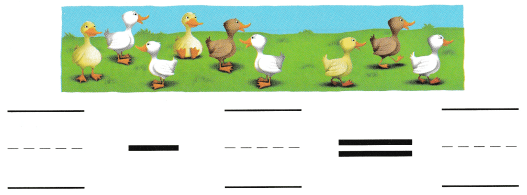Explanation:
There are nine ducks.
Three ducks leave,
9 – 3 = 6
6 ducks are left

Question 5.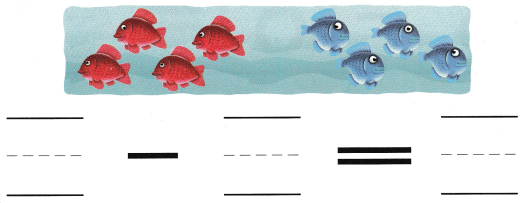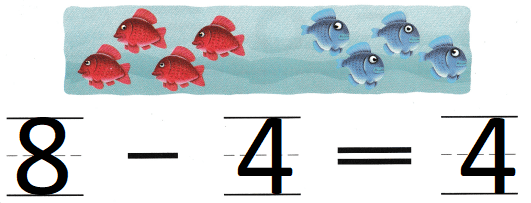Explanation:
There are eight fish. Four of the fish are blue.
The rest are red.
8 fish are there in all
8 – 4 = 4
so, 4 fish are red.

Question 6.
Texas Test Prep